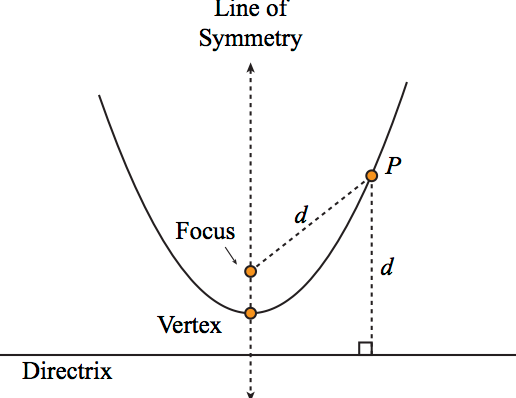#### Find the equation of the hyperbola whose directrix is 2x + y = 1, focus (1, 2) and eccentricityOption 1) 7x2 - 2y2 + 12xy - 2x + 14y - 22 = 0 Option 2) 7x2 - 2y2 + 2xy - 2x + 14y - 22 = 0 Option 3) 7x2 - 2y2 + xy - 14x + 2y - 22 = 0 Option 4) none of the aboveEccentricity -

The ratio of a distance of point from focus to distance from fixed line.

- wherein

It is donated by.

&

Directrix -

The fixed straight line of a conic section.

- wherein&

Focus -

The fixed point of a conic section

- whereinOption 1)

7x2 - 2y2 + 12xy - 2x + 14y - 22 = 0

This solution is correct

Option 2)

7x2 - 2y2 + 2xy - 2x + 14y - 22 = 0

This solution is incorrect

Option 3)

7x2 - 2y2 + xy - 14x + 2y - 22 = 0

This solution is incorrect

Option 4)

none of the above

This solution is incorrect